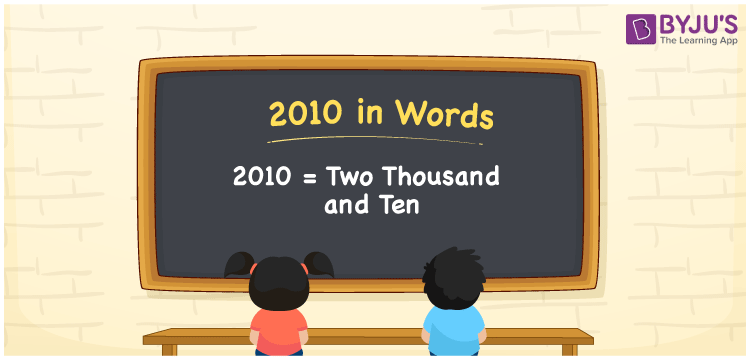# 2010 in Words

We can write 2010 in words as Two thousand and ten. The word form of 2010 is the number name for 2010, that is Two thousand and ten. This name is used to express a certain amount or quantity, equivalent to 2010. Suppose you purchased a shirt that costs Rs. 2010, you could say, “I purchased a shirt worth Two thousand and ten rupees”. Let’s understand how to write the cardinal number 2010 in words here, in this article.

 2010 in words Two thousand and ten Two thousand and ten in Numbers 2010

## 2010 in English Words

We generally write numbers in words using the English alphabet. Thus, we can spell 2010 in English as “Two thousand and ten”.## How to Write 2010 in Words?

In this section, you will learn the conversion of the number 2010 into words with the help of a four-column place value chart since 2010 has four digits.

 Thousands Hundreds Tens Ones 2 0 1 0

Here, ones = 0, tens = 1, hundreds = 0, thousands = 2

Now, by expanding these digits, we get;

2 × Thousand + 0 × Hundred + 1 × Ten + 0 × One

= 2 × 1000 + 0 × 100 + 1 × 10 + 0 × 1

= 2000 + 10

= Two thousand + Ten

= Two thousand and ten

Therefore, 2010 in words = Two thousand and ten.

As we know, 2010 is a natural number that precedes 2011 and succeeds 2009.

2010 in words – Two thousand and ten

Is 2010 an odd number? – No

Is 2010 an even number? – Yes

Is 2010 a perfect square number? – No

Is 2010 a perfect cube number? – No

Is 2010 a prime number? – No

Is 2010 a composite number? – Yes

## Frequently Asked Questions on 2010 in Words

Q1

### How do you write the year 2010 in words?

We can write the year 2010 in words as Two thousand and ten.
Q2

### What is the number name of 2010?

Two thousand and ten is the number name of 2010.
Q3

### Find the value of two thousand and ten plus Two thousand and twenty.

Two thousand and ten plus Two thousand and twenty = 2010 + 2020 = 4030 Therefore, the value of two thousand and ten plus Two thousand and twenty is Four thousand and thirty.